# CART Classification (R)

## Decision Trees¶

Decision trees are a collection of predictive analytic techniques that use tree-like graphs for predicting the response variable. One such method is CHAID explained in a previous blog. The most popular decision tree method is the CART or the Classification and regression trees. Decision trees partition the data set into mutually exclusive and exhaustive subsets, which results in the splitting of the original data resembling a tree-like structure.

## CART Classification¶

We can use the CART method for regression and classification. In this blog, I will go through the CART classification in detail using the titanic example. Any decision tree model consists of 5 steps:
1. Start with the complete training data in the root node.
2. Decide on a splitting criterion
3. Split the data into two or more subsets based on the above metric
4. Using independent variables, repeat step 3 for each of the subsets of the data until
--(a) All the dependent variables are exhausted, or they are not statistically significant at alpha
--(b) We meet the stopping criteria
5. Generate business rules for the terminal nodes (nodes without any branches) of the tree

### Step 1: Start with complete data¶

The data used in this blog is the same as the used in other classification posts, i.e. the Titanic dataset from Kaggle. In this problem, we have to identify what types of people had a higher chance of survival. A sample of the data is shown below. A basic EDA is performed in the blog on Logistic regression.

Titanic dataset
Survived Pclass Sex Age SibSp Parch Fare Embarked
I 3 female 14 1 0 11.2417 C
O 2 male 21 1 0 11.5000 S
I 2 female 24 0 2 14.5000 S
I 2 female 29 0 0 10.5000 S
O 1 male 27 0 2 211.5000 C

### Step 2: Decide on a splitting criterion¶

In CHAID bases classification, we used p-value from hypothesis tests as the splitting criterion. In CART, we will use impurity metrics like Gini Index and Entropy.

#### Gini Index¶

It is defined mathematically as: $$Gini\,Index = \sum_{i=1}^k \sum_{j=1,i\neq 1}^k P(C_i|t)\times P(C_j|t)$$ where $P(C_i|t)$ = Proportion of observations belonging to class $C_i$ in node t. For a binary classification,

$Gini\,Index = 1 - \sum_{i=1}^k P(C_i|t)^2$

Gini Index is a measure of total variance across the classes. It has a small value if all the $P(C_i|t)$ are close to zero or one. For this reason, the Gini index is a measure of node purity. A small value indicates that a node contains observations from a single class predominantly.

#### Entropy¶

An alternative to the Gini index is entropy, given by entropy: $$Entropy = - \sum_{i=1}^k P(C_i|t)\times log[P(C_i|t]$$ Like the Gini index, the entropy is a small value if the node is pure. The Gini index and the entropy are quite similar numerically.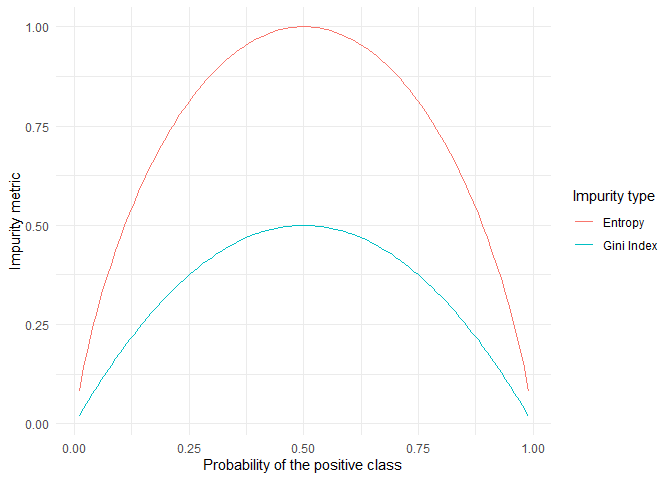For this blog, I want to consider the Gini index as my impurity measure. The aim is to minimise the Gini Index as much as possible.

### Step 3. Split the data into two or more subsets based on the above metric¶

The Gini impurity in the base node (complete data) is

##  0.472365


The impurity of the overall data is 0.47. Let us look at the purity if we split the data based on various features.

#### Gender:¶

If we split based on gender, the male and female branches individually have lower impurity (Gini index) than the base node. I have built the decision tree as the total weighted Gini index after splitting is lesser than the Gini index of the overall data.

Gender
Var1 I O proportion.of.obs child.gini.index
female 231 81 0.3509561 0.3844305
male 109 468 0.6490439 0.3064437
##  "The total weighted impurity after the split is: 0.3338"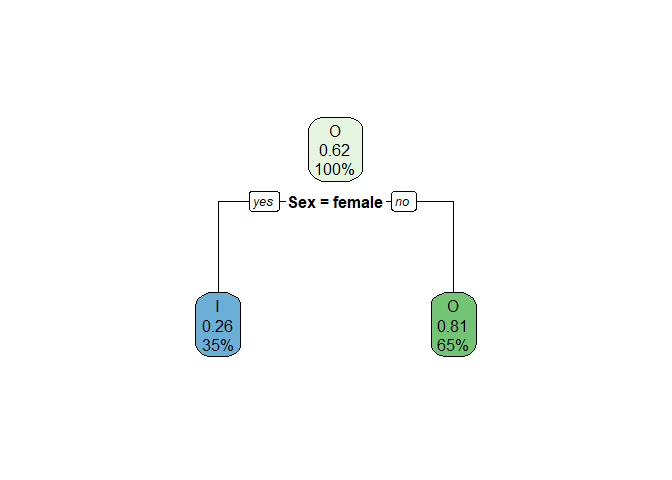#### Passenger class¶

Similarly, for passenger class, the three categories (if we split into three branches) have an impurity as follows:

Passenger Class
Var1 I O proportion.of.obs child.gini.index
1 134 80 0.2407199 0.4681632
2 87 97 0.2069741 0.4985232
3 119 372 0.5523060 0.3672459

In CART, we can split the data into two branches only. By combining passenger class 1 and passenger class 2 into one category, we will have the below Gini impurities. I have built the decision tree as the total weighted Gini index after splitting is lesser than the Gini index of the overall data.

Passenger Class
Var1 I O proportion.of.obs child.gini.index
3rd Class 119 372 0.552306 0.3672459
First and second Class 221 177 0.447694 0.4938890
##  "The total weighted impurity after the split is: 0.423943259996532"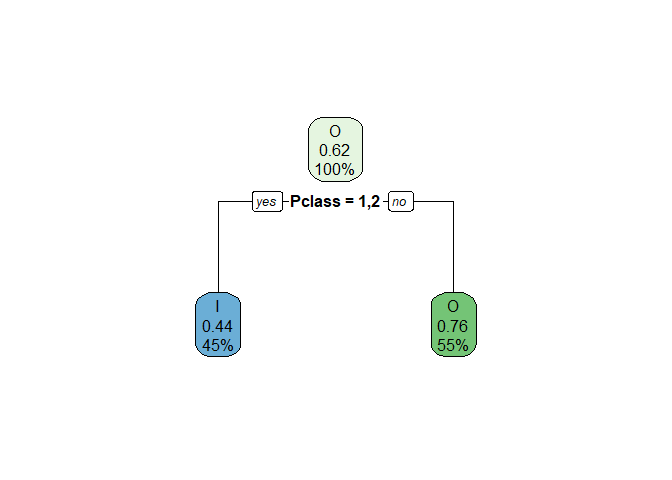#### Number of siblings¶

The number of siblings is a categorical variable in this problem as the probability of survival is not linearly dependent on the number of siblings. According to intuition, people with one or two siblings will have a higher rate of survival as they will take care of each other. The impurity for each class is:

No of siblings
Var1 I O proportion.of.obs child.gini.index
0 208 398 0.6816648 0.4508490
1 112 97 0.2350956 0.4974245
2 13 15 0.0314961 0.4974490
3 4 12 0.0179978 0.3750000
4 3 15 0.0202475 0.2777778
5 0 5 0.0056243 0.0000000
8 0 7 0.0078740 0.0000000

By combining the number of siblings of 1 and 2 into one category and all others into another group, we will have the following Gini index:

No of siblings
Var1 I O proportion.of.obs child.gini.index
One or two siblings 125 112 0.2665917 0.4984956
others 215 437 0.7334083 0.4420330
##  "The total weighted impurity after the split is: 0.457085468377958"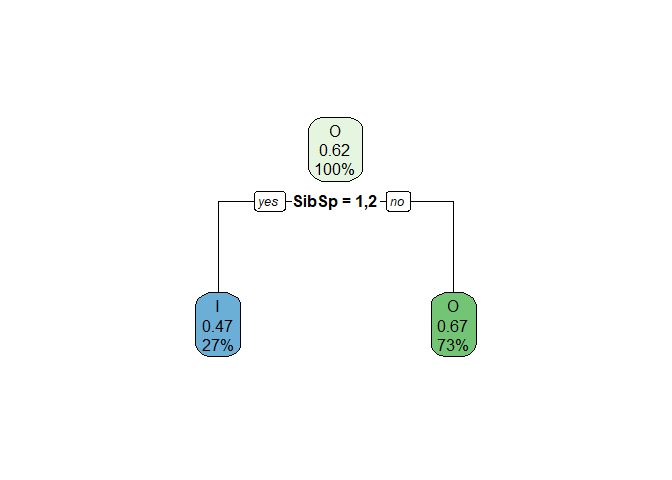#### Number of parents/children¶

Just like the number of siblings, the number of parents/children of a person is also a categorical variable. The Gini index among the categories are:

Parch
Var1 I O proportion.of.obs child.gini.index
0 231 445 0.7604049 0.4498923
1 65 53 0.1327334 0.4948291
2 40 40 0.0899888 0.5000000
3 3 2 0.0056243 0.4800000
4 0 4 0.0044994 0.0000000
5 1 4 0.0056243 0.3200000
6 0 1 0.0011249 0.0000000

By combining the number of parents/siblings of 1, 2 and 3 into one group and others into another group, we will have the following impurity:

Parch
Var1 I O proportion.of.obs child.gini.index
1,2&3 108 95 0.2283465 0.4979495
others 232 454 0.7716535 0.4476366
##  "The total weighted impurity after the split is: 0.459125378001722"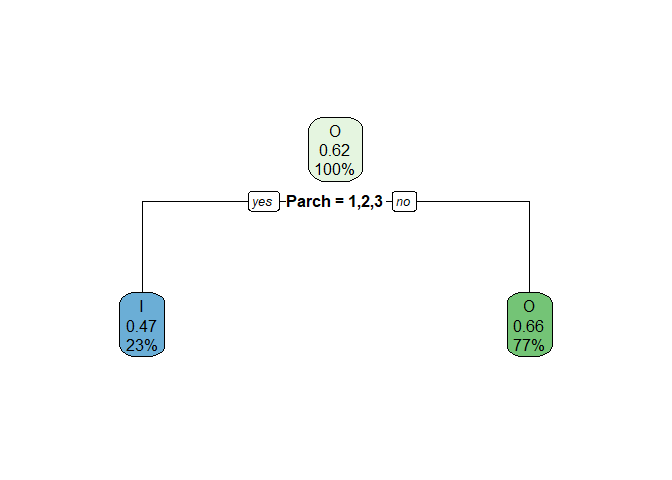#### Embarked location¶

There are three locations where the passengers have embarked. The Gini index within each of the location is:

Embarked location
Var1 I O proportion.of.obs child.gini.index
C 93 75 0.1889764 0.4942602
Q 30 47 0.0866142 0.4756283
S 217 427 0.7244094 0.4468336

Combining Q and S into one category, we have:

Embarked location
Var1 I O proportion.of.obs child.gini.index
C 93 75 0.1889764 0.4942602
Q&S 247 474 0.8110236 0.4504377
##  "The total weighted impurity after the split is: 0.458719142423424"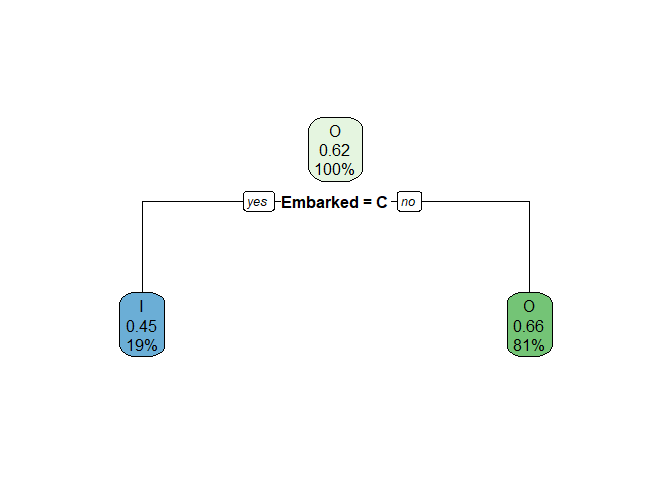#### Age¶

Age is a continuous variable, so we have to find the ideal age to split the data along with the impurity metrics. To find the ideal cutoff, I have plotted the weighted gini index for split based on different ages.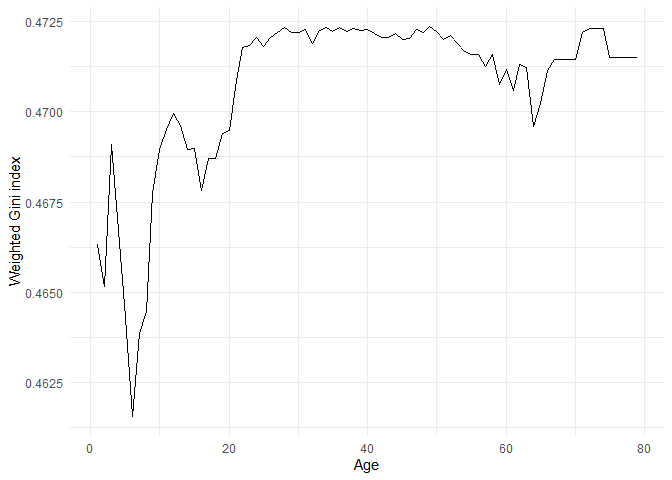We can observe the minimum Gini index when the data is split at Age=7 years.

Age
Age_class survived_prob proportion.of.obs child.gini.index
<7 0.6470588 0.0573678 0.4567474
>=7 0.3663484 0.9426322 0.4642745
##  "The total weighted impurity after the split is: 0.46"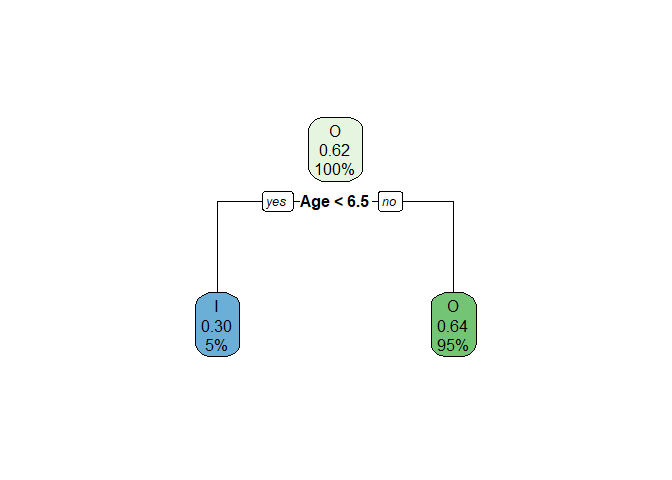#### Fare¶

Similar to age, fare is a continuous variable and we have to find the optimal fare to split first.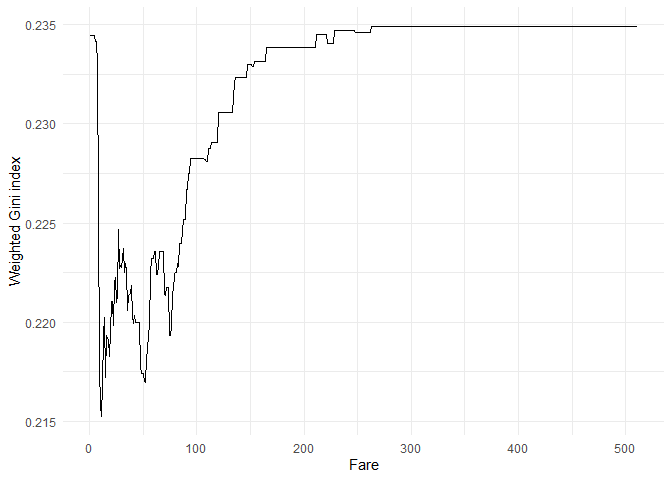The optimal fare is 11. The impurity metrics for this split are:

Fare
Fare_class survived_prob proportion.of.obs child.gini.index
<=11 0.2087912 0.4094488 0.3303949
>11 0.5028571 0.5905512 0.4999837

#### Conclusion¶

Among all the factors above, Gender has the least Gini Index of 0.3338. So the initial split of the data would be on Gender.

### Step 4: Repeat step 3 until stopping criterion¶

After splitting the data based on gender, we will get two data sets. We should perform a similar analysis to step 2 to split the data further, and so on. The splitting should stop when we meet the stopping criterion. Different stopping criterion for CART classification trees are:
1. The number of cases in the node is less than some pre-specified limit.
2. The purity of the node is more than some pre-specified threshold.
3. Depth of the node is more than some pre-specified threshold.
4. Predictor values for all records are identical

In this blog, I want to give a maximum depth of trees as 3. In the machine learning blogs, I will go deeper into how to select ideal stopping criterion. For max depth=3, we get the following decision tree: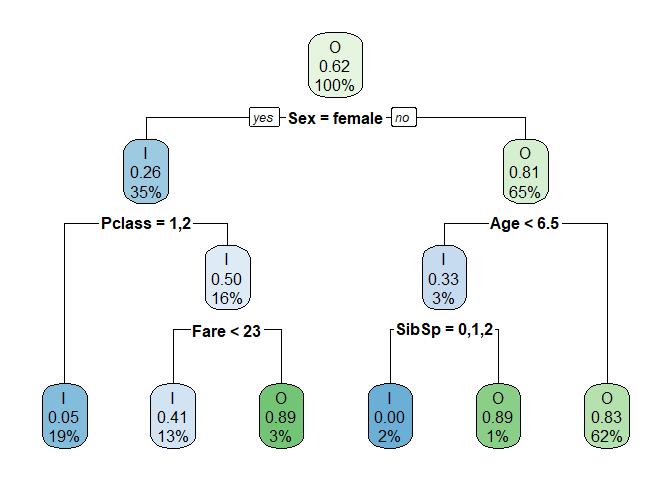### Step 5: Making business rules¶

From the above tree, I can see generate the following rules:
1. A female passenger has a higher probability of surviving unless she is travelling in third class with a fare less than 23.
2. A male passenger has a lower likelihood of surviving unless he is a child of age less than six years and with up to two siblings.

Using this decision tree, we can observe how sociological factors like gender, age and monetary status affected the decisions on who would get into a lifeboat at a crucial time during the titanic disaster.